# Chapter 4 Quadrilateral Equations NCERT Exemplar Solutions Exercise 4.4 Class 10 Maths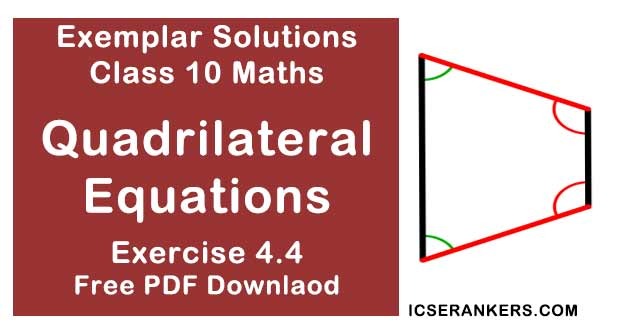Chapter Name NCERT Maths Exemplar Solutions for Chapter 4 Quadrilateral Equations Exercise 4.4 Book Name NCERT Exemplar for Class 10 Maths Other Exercises Exercise 4.1Exercise 4.2Exercise 4.3 Related Study NCERT Solutions for Class 10 Maths

### Exercise 4.4 Solutions

1. Find whether the following equations have real roots. If real roots exist, find them.
(i) 8x2 + 2x - 3 = 0
(ii) -2x2 + 3x + 2 = 0
(iii) 5x2 - 2x - 10 = 0
(iv) 1/(2x - 3) + 1/(x - 5) = 0
(v) x2 + 5√5x - 70 = 0

Solution

For real roots of quadratic equation x2 + bx + c = 0,
Also, b2 - 4ac > 0
(i) The given equation is 8x2 + 2x - 3 = 0
Discriminant (D) = b2 - 4ac
D = (2)2 - 4(8)(-3)  [where, a = 8, b = 2, c = -3]
⇒ D = 4 + 96
⇒ D = 100
As D > 0, so, roots are real.
Now,
Discriminant = 100
So, roots are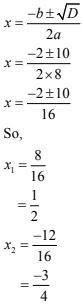So, the roots of the given equation are 1/2 and -3/4.

(ii) -2x2 + 3x + 2 = 0
Discriminant D = b2 - 4ac
D = (3)2 - 4(-2)(2)  [where, a = –2, b = 3, c = 2]
⇒ D = 9 + 16
⇒ D = 25 > 0
So, the given equation has real and distinct roots.
Now,
D = 25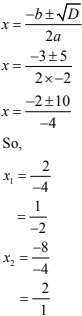So, the roots of the given equation are 2 and -1/2.

(iii) 5x2 - 2x - 10 = 0
Discriminant D = b2 - 4ac
D  = (-2)2 - 4(5)(-10)  [where, a = 5, b = -2, c = -10]
⇒ D = 4 + 200
⇒ D = 204 > 0
So, the roots of the given equation are real and distinct.
Now,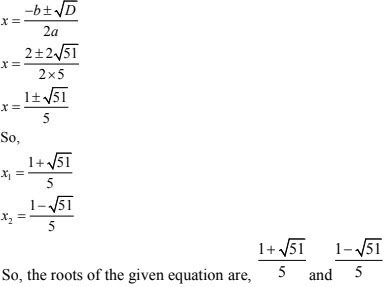(iv) 1/(2x - 3) + 1/(x - 5) = 1
⇒ 2x2 - 10x - 3x + 15 = x - 5 + 2x - 3
⇒ 2x2 - 13x + 15 = 3x - 8
⇒ 2x2 - 13x + 15 - 3x + 8 = 0
⇒ 2x2 - 16x + 23 = 0
Now,
D = b2 - 4ac
⇒ D = (-16)2 - 4(2)(23)  [where, a = 2 , b = -16, c = 23]
⇒ D = 256 - 184
⇒ D = 72 > 0
So, the roots are,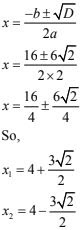So, these are the roots of the given quadratic equation.

(v) x2 + 5√5x - 70 = 0
D = b2 - 4ac
⇒ D = 25 × 5 + 280
⇒ D = 125 + 280
⇒ D = 405 > 0
So, the roots of the given equation are real and distinct.
For roots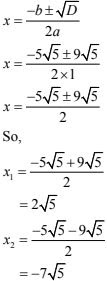Above are the roots of the given quadratic equation.

2. Find a natural number whose square diminished by 84 is equal to thrice of 8 more than the given number.

Solution

Let us take the natural number = 'x'.
Now from the question,
We have the equation,
x2 - 84 = 3(x + 8)
⇒ x2 - 84 = 3x + 24
⇒ x2 - 3x - 84 - 24 = 0
⇒ x2 - 3x - 108 = 0
⇒ x2 - 12x + 9x - 108 = 0
⇒ x(x - 12) + 9(x - 12) = 0
⇒ (x + 9)(x - 12) = 0
⇒ x = -9 and x = 12
As, natural numbers cannot be negative.
The number is  12.

3. A natural number, when increased by 12, equals 160 times its reciprocal. Find the number.

Solution

Let us take the natural number = x
When the number increased by 12 = x + 12
Reciprocal of the number = 1/x
From the question,
x + 12 = 160 times of reciprocal of x
⇒ x + 12 = 160/ x
⇒ x( x + 12 ) = 160
⇒ x2 + 12x - 160 = 0
⇒ x2 + 20x - 8x - 160 = 0
⇒ x(x + 20) - 8(x + 20) = 0
⇒ (x + 20)(x - 8) = 0
⇒ x + 20 = 0 or x - 8 = 0
⇒ x = -20 or x = 8
As, natural numbers cannot be negative.
The required number = x = 8

4. A train, travelling at a uniform speed for 360 km, would have taken 48 minutes less to travel the same distance if its speed were 5 km/h more. Find the original speed of the train.

Solution

Let us take the original speed of train = x km/h
We have,
Time = Distance/Speed
From the question, we have,
Time taken by train = 360/x hour
And, Time taken by train its speed increase 5 km/h = 360/( x + 5)
It is given that,
Time taken by train in first – time taken by train in 2nd case = 48 min = 48/60 hour
360/x + 360/(x + 5) = 48/60
⇒ 360(1/x + 1/(x+ 5)) = 4/5
⇒ 450 × 5 = x2 + 5x
⇒ x2 + 5x - 2250 = 0
On solving,
x = -50, 45
But,
x ≠ -50 as speed cannot be negative
Therefore,
x = 45 km/h
So, original speed of train = 45 km/h

5. If Zeba were younger by 5 years than what she really is, then the square of her age (in years) would have been 11 more than five times her actual age. What is her age now?

Solution

Let us take Zeba's age = x
From the question, we have,
(x - 5)2 = 11 + 5x
⇒ x2 + 25 - 10x = 11 + 5x
⇒ x2 - 15x + 14 = 0
⇒ x2 - 14x - x + 14 = 0
⇒ x(x - 14) - 1(x - 14) = 0
⇒ x = 1 or x = 14
We neglect 1 as 5 years younger than 1 is not possible.
So, Zeba's present age = 14 years.

6. At present Asha’s age (in years) is 2 more than the square of her daughter Nisha’sage. When Nisha grows to her mother’s present age, Asha’s age would be one year less than 10 times the present age of Nisha. Find the present ages of both Asha and Nisha.

Solution

Taking the present age of Asha = x years
The present age of her daughter Nisha = y years
At Present, Asha's age, x = (y)2 + 2
Age of Nisha will be equal to age of her mother (x) after no of years = Age of Mother - Age of Daughter
= (x - y)
= (y2 + 2 -y)
= (y2 - y + 2) years
∴ Age of (Nisha) daughter after (y2 - y + 2) years = y2 - y + 2 + y = (y2 + 2) years
Age of Asha (mother) after (y2 - y + 2) years
= x + y2 - y + 2
= y2 + 2 + y2 - y + 2 [From (i)]
= 2y2 - y + 4 years
After (y2 - y - 2) years, age of Asha = 2y2 - y + 4 = 10y - 1
2y2 – y – 10y + 5 = 0
⇒ 2y2 – 11y + 5 = 0
⇒ 2y2 – 10y – 1y + 5 = 0
⇒ 2y(y – 5) – 1(y – 5) = 0
⇒ (y – 5) (2y – 1) = 0
⇒ y – 5 = 0 or 2y – 1 = 0
⇒ y = 5 or y = years
From (i), we have
x = y2 + 2
Putting y = 5, we have
x = (5)2 + 2 = 25 + 2 = 27
Mother’s age can never be years, so it is not taken.
So, the ages of Asha and Nisha are 27 years and 5 years respectively.

7. In the centre of a rectangular lawn of dimensions 50 m × 40 m, a rectangular pond has to be constructed so that the area of the grass surrounding the pond would be 1184 m2  [see Fig]. Find the length and breadth of the pond.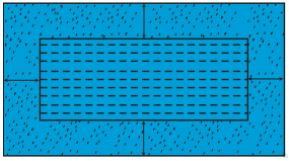Solution

In the figure both Pond and lawn are rectangular.
Pond is inside the lawn.
Let the length of pond = (50 – 2x) m
The breadth of pond = (40 – 2x) m
Also,
Area of grass around the pond = 1184 m2
Area of Lawn – Area of Pond = 1184
50 × 40 – (50 – 2x) (40 – 2x) = 1184
⇒ 2000 – (2000 – 100x – 80x + 4x2 ) – 1184 = 0
⇒ 2000 – (2000 – 180x + 4x2 ) – 1184 = 0
⇒ 2000 – 2000 + 180x – 4x2 – 1184 = 0
⇒ 4x2 - 180x + 1184 = 0
⇒ x2 - 45x + 296 = 0
⇒ x2 - 37x - 8x + 296 = 0
⇒ x(x - 37) - 8(x - 37) = 0
⇒ (x - 37)(x - 8) = 0
⇒ x - 37 = 0
⇒ x = 37
or,
x - 8 = 0
⇒ x = 8
When x = 37, then
The length of pond = 50 - 2 × 37
= 50 - 74 = -24 m
Length cannot be negative. So, x = 37 is not taken.
When x = 8, then
The length of pond = 50 – 2x
= 50 – 2x × 8
= 50 – 16
= 34 m
And the breadth of the pond = 40 – 2x
= 40 – 2 × 8
= 40 – 16
= 24 m
Hence, the length and breadth of the pond are 34 m and 24 m respectively.

8. At t minutes past 2 p.m., the time needed by the minute hand of a clock to show 3 p.m. was found to be 3 minutes less than  t2 /4 minutes . Find t.

Solution
It is given that total time taken by min. hand from 2 p.m. to 3 p.m. is 60 min.
After t min past 2 p.m. the time needed by min. hand of a clock to show 3 p.m. is given by 3 min less than t2 /4 min.
t + (t2 /4 - 3) = 60
⇒ 4t + t2 - 12 = 240
⇒ t2 + 4t - 252 = 0
⇒ t2 + 18t - 14t - 252 = 0
⇒ t(t + 18) - 14(t + 18) = 0
⇒ (t + 18)(t - 14) = 0
⇒ t + 18 = 0 or t - 14 = 0
⇒ t = -18 or t = 14 min.
Being, negative value, t = -18 is not taken .
So, t = 14 min.